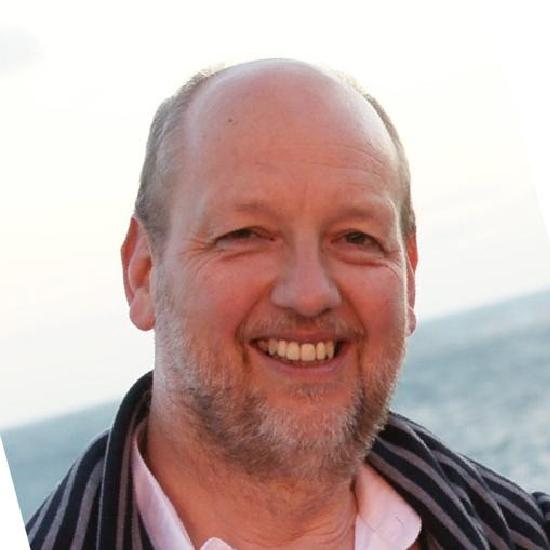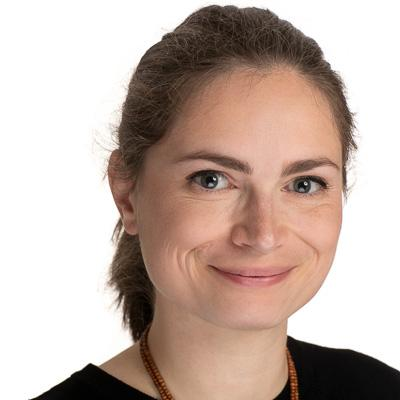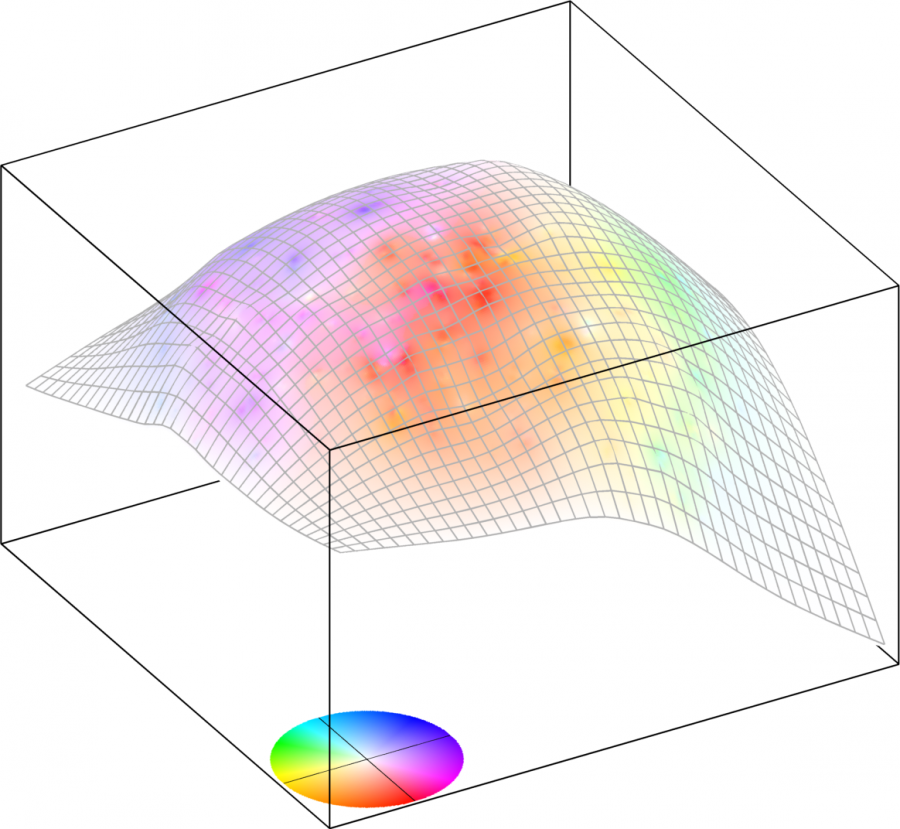# DNA faculty working on optimizationRonny Bergmann is working on optimization on Riemannian manifolds. Often, when working with nonlinear data many tasks are phrased as optimisation problems where the cost function is defined on a Riemannian manifold. Optimization methods on Riemannian manifolds take the geometry into account and hence stay intrinsicly on the manifold. This way one avoids to work in the embedding on a constraint problem, but obtains an unconstraint problem. A main research topic is to develop fast and efficient algorithms to compute a minimizer for cost functions on manifolds, especially for high-dimensional manifolds and nonsmooth cost functions. One area of applications is manifold-valued image processing, where the data is a 2D image or 3D voxel data, where every pixel is a value on a manifold, for example in DT-MRI, every pixel or voxel is a symmetic positive definite matrix. Tasks like denoising, inpainting or labelling can then be phrased as optimization tasks on (power) manifolds. The developed algorithms and considered manifolds are implemented in Julia and available in the two packages Manifolds.jl and Manopt.jl. Ronny also works on multivariate anisotropic Fourier and wavelet transforms and their application in image processing.Markus Grasmair is working on inverse problems and image processing. In particular, he is interested in the analysis of variational regularisation methods based on non-quadratic Tikhonov regularisation, specifically for sparsity enforcing models. Within image processing, he mainly works on methods related to total variation regularisation and the Mumford-Shah model, which are used to treat problems like denoising and deblurring of images, but also motion estimation and computation of the optical flow. A common theme in all these problems is that they lead to non-smooth optimisation problems in Hilbert spaces that are tackled with ideas from (generalised, abstract) convex analysis.Dietmar Hömberg works on optimal control problems with an emphasis on application to real world problems. He studies multi-field problems coupling electromagnetics, heat transfer, and possibly adding further equations to describe microstructural changes caused by temperature evolution. Applications range from the heat treatment and welding of steel to additive manufacturing and medical applications in cancer therapy. Another area of research is topology optimization based on phasefield regularization. Recent results in this field are related to the creation of graded materials or multiscale structures.Elisabeth Köbis is working on set optimization, which is a modern and expanding branch of mathematics that deals with optimization problems where the objective map and possibly the constraint mappings are set-valued maps acting between certain spaces. If the objective mapping is single-valued, then this field recovers the concept of vector/multiobjective optimization. In a finite-dimensional setting, one speaks of multiobjective optimization. Here, several objective functions, which are usually conflicting, are minimized in parallel. Almost any real-world application in mathematical optimization has multiple conflicting criteria. For example, the problem of choosing a portfolio in financial mathematics, where the risk is to be minimized while the returns should be maximized simultaneously, can be regarded as a problem including conflicting goals. In a more abstract setting, when the space is infinite dimensional, one speaks of vector optimization. Here, one is concerned with optimization in functional spaces. In relation with vector optimization problems, one is often concerned with vector variational inequalities, which are regarded as a powerful tool to study vector optimization problems. For example, one can show the equivalence between optimal solutions of vector optimization problems with differentiable convex objective function and solutions of vector variational inequalities (of a so-called Minty type). Elisabeth is also working on programming under uncertainty as an application of set optimization, where one follows a so-called robust approach, which is set-based and non-probabilistic. Here, one assumes that all possible objective function values for each feasible solution are comprised in a set, and one looks for the minimal solution.

## Optimization right barVector field depicting the movement of the endodermal cells of a zebra fish embryo on the surface of the embryo's yolk. From the paper: Martin Bauer, Markus Grasmair, and Clemens Kirisits. Optical Flow on Moving Manifolds. SIAM Journal of Imaging Sciences. vol. 8 (1), 2015.